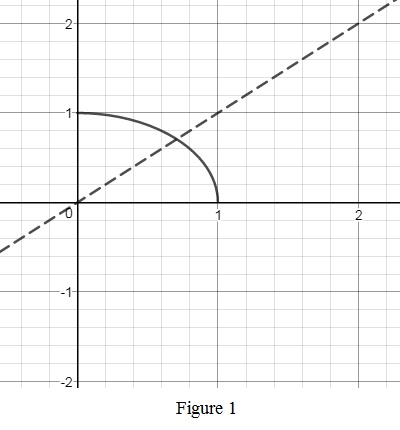# The inverse of the function f ( x ) = 1 − x 2 , 0 ≤ x ≤ 1 ; how f − 1 is related to f .### Single Variable Calculus: Concepts...

4th Edition
James Stewart
Publisher: Cengage Learning
ISBN: 9781337687805### Single Variable Calculus: Concepts...

4th Edition
James Stewart
Publisher: Cengage Learning
ISBN: 9781337687805

#### Solutions

Chapter 1.6, Problem 31E

(a)

To determine

## To find: The inverse of the function f(x)=1−x2,0≤x≤1 ; how f−1 is related to f.

Expert Solution

The inverse of the function f(x)=1x2,0x1 is f1(x)=1x2 and f1=f .

### Explanation of Solution

Let the given function as y=1x2 .

Solve this equation for x as follows.

y=1x2y2=1x21y2=x2x=±1y2

Interchange x and y and obtain the inverse function, y=±1x2 . Since the given function f(x)=4x+3 has the restriction as 0x1 , ignore the negative root of x.

Thus, the required inverse function is f1(x)=1x2 .

Observe that the given function and its inverse are the same. That is f1=f .

(b)

To determine

Expert Solution

### Explanation of Solution

The graphs of f(x)=1x2,0x1 is shown below in Figure 1.From Figure 1, it is observed that the graph of f(x)=1x2,0x1 is a reflection of the graph f1(x)=1x2 about the line y=x . Notice that the graphs of f1(x) and f(x) are same.

### Have a homework question?

Subscribe to bartleby learn! Ask subject matter experts 30 homework questions each month. Plus, you’ll have access to millions of step-by-step textbook answers!